direcs  2012-09-30

Responsible for getting all data from all sensors - except laserscanners. More...

`#include <sensorThread.h>`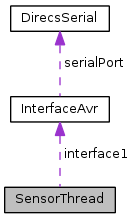[legend]

List of all members.

## Public Slots

bool resetDrivenDistance (int sensor)
void setCompassState (bool state)
void setRobotState (bool state)
void setSimulationMode (bool state)

## Signals

void compassDataComplete (float x, float y, float z, float heading)
void contactAlarm (char position, bool state)
void heartbeat (unsigned char state)
void message (QString text, bool CR=true, bool sayIt=false, bool addTimestamp=true)
void sendNetworkString (QString text)
void sensorDataComplete ()
void systemerror (int errorlevel)

## Public Member Functions

int convertToDistance (int sensorValue)
int convertToSensorValue (int distance)
int getCompassValue (unsigned char axis)
int getContactValue (int contact)
int getDistance (int sensor)
int getDrivenDistance (int sensor)
int getHeartbeatValue ()
int getIrSensorValue (int sensor)
int getMAmpere (int motor)
int getMotorSensorValue (int sensor)
int getUsSensorValue (int sensor)
float getVoltage (int sensor)
virtual void run ()
void stop ()

## Private Member Functions

float convertToDegree (int sensorValue)
float convertToVolt (int sensor)

## Private Attributes

QString className
bool compassState
char contactValue [CONTACTARRAYSIZE]
int drivenDistance [DRIVENDISTANCEARRAYSIZE]
int heartbeatValue 
InterfaceAvrinterface1
int iRDistance [IRSENSORARRAYSIZE]
int iRSensorValue [SENSOR8+1]
int motorSensorValue [MOTORSENSORARRAYSIZE]
QMutex * mutex
this will contain the name of this class at runtime for debug messages
bool robotState
bool simulationMode
volatile bool stopped
int usSensorValue [USSENSORARRAYSIZE]
int voltageSensorValue 
float xAxis
float yAxis
float zAxis

## Static Private Attributes

static const char BOTTOM = 3
static const unsigned char CONTACT1 = 0
static const unsigned char CONTACT2 = 1
static const unsigned char CONTACT3 = 2
static const unsigned char CONTACT4 = 3
static const unsigned char CONTACTARRAYSIZE = 4
static const unsigned char CONVERSIONFACTORMOTORSENSOR = 29
static const float CONVERSIONFACTORVOLTAGESENSOR1 = 243.75
static const float CONVERSIONFACTORVOLTAGESENSOR2 = 162.50
static const short int DRIVENDISTANCE1 = 0
static const short int DRIVENDISTANCE2 = 1
static const unsigned char DRIVENDISTANCEARRAYSIZE = 2
static const unsigned char GREEN = 2
static const unsigned char IRSENSORARRAYSIZE = 40
static const unsigned char LEDOFF = 0
static const char LEFT = 0
static const int MAXIMUMPLOTHEARTBEAT = 5
static const short int MOTORSENSOR1 = 0
static const short int MOTORSENSOR2 = 1
static const short int MOTORSENSOR3 = 2
static const short int MOTORSENSOR4 = 3
static const unsigned char MOTORSENSORARRAYSIZE = 4
static const bool OFF = false
static const bool ON = true
static const unsigned char READ_AXIS_X = 61
static const unsigned char READ_AXIS_Y = 62
static const unsigned char READ_AXIS_Z = 63
static const unsigned char READ_CONTACT1 = 70
static const unsigned char READ_CONTACT2 = 71
static const unsigned char READ_CONTACT3 = 72
static const unsigned char READ_CONTACT4 = 73
static const unsigned char READ_MOTOR_DISTANCE1 = 30
static const unsigned char READ_MOTOR_DISTANCE2 = 31
static const unsigned char READ_MOTOR_SENSOR1 = 28
static const unsigned char READ_MOTOR_SENSOR2 = 29
static const unsigned char READ_SENSOR_1 = 1
static const unsigned char READ_SENSOR_16 = 16
static const unsigned char READ_SENSOR_2 = 2
static const unsigned char READ_SENSOR_3 = 3
static const unsigned char READ_SENSOR_4 = 4
static const unsigned char READ_SENSOR_5 = 5
static const unsigned char READ_SENSOR_6 = 6
static const unsigned char READ_SENSOR_7 = 7
static const unsigned char READ_SENSOR_8 = 8
static const unsigned char RED = 1
static const short int RESET_MOTOR_DISTANCE1 = 32
static const short int RESET_MOTOR_DISTANCE2 = 33
static const char RIGHT = 1
static const short int SENSOR1 = 1
static const short int SENSOR16 = 256
static const short int SENSOR2 = 2
static const short int SENSOR3 = 4
static const short int SENSOR4 = 8
static const short int SENSOR5 = 16
static const short int SENSOR6 = 32
static const short int SENSOR7 = 64
static const short int SENSOR8 = 128
static const unsigned long THREADSLEEPTIME = 1000
static const char TOP = 2
static const short int ULTRASONICSENSOR1 = 0
static const unsigned char USSENSORARRAYSIZE = 1
theoratically 5 Volt for heartbeat "high"
static const short int VOLTAGESENSOR1 = 0
static const short int VOLTAGESENSOR2 = 1
static const short int XAXIS = 0
static const short int YAXIS = 1
static const short int ZAXIS = 2

## Detailed Description

Responsible for getting all data from all sensors - except laserscanners.

Definition at line 39 of file sensorThread.h.

## Constructor & Destructor Documentation

Definition at line 23 of file sensorThread.cpp.

Definition at line 145 of file sensorThread.cpp.

Here is the call graph for this function: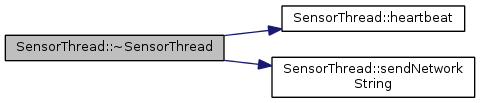## Member Function Documentation

private

Translates the x and y data from the 3D compass into a single orientation reading.

Definition at line 1054 of file sensorThread.cpp.

 void SensorThread::compassDataComplete ( float x, float y, float z, float heading )
signal

This signal is emitted when all compass values were read.

Parameters:
 x is the x axis value y is the y axis value z is the z axis value heading is the heading of the compass
 void SensorThread::contactAlarm ( char position, bool state )
signal

This signal is emitted when a contact value is read. The result (an alarm) will be displayed immediately in the camera live image.

Direcs::showContactAlarm()
 float SensorThread::convertToDegree ( int sensorValue )
private

Converts the compass sensor value (16 bit integer 2's complement format) to degrees (0 to 360).

Parameters:
 sensorValue
Returns:
The given value in degrees (0 to 360).

Definition at line 1033 of file sensorThread.cpp.

Here is the caller graph for this function: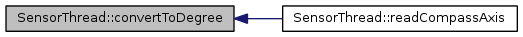int SensorThread::convertToDistance ( int sensorValue )

Converts a infrared sensor value to a distance in cm.

Parameters:
 sensorValue is the measured sensor value.
Returns:
The distance in cm.
convertToSensorValue()

Definition at line 627 of file sensorThread.cpp.

Here is the caller graph for this function: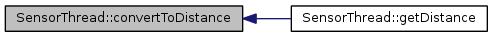int SensorThread::convertToSensorValue ( int distance )

Converts a distance in cm to an infrared sensor value.

Parameters:
 distance is the distance in cm.
Returns:
The sensor value.
convertToDistance()

Definition at line 649 of file sensorThread.cpp.

Here is the caller graph for this function: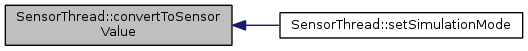float SensorThread::convertToVolt ( int sensor )
private

Converts a voltage sensor value to a value in Volt (V).

Parameters:
 sensor is the sensor number.
Returns:
The voltage in Volt.

Definition at line 1083 of file sensorThread.cpp.

Here is the caller graph for this function: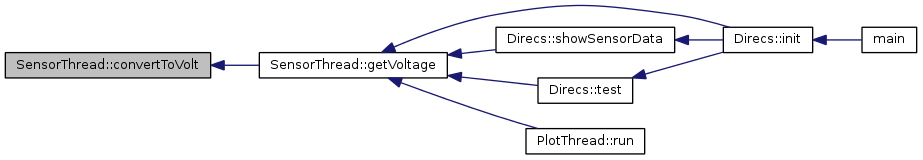int SensorThread::getCompassValue ( unsigned char axis )
Parameters:
 axis is the x, y or z axis.
Returns:
The measured x, y and z value from the 3D magnetic compass module (micromag3).
 int SensorThread::getContactValue ( int contact )
Parameters:
 contact is the contact number.
Returns:
The 'measured' contact value. 0 if OFF; unequal to 0 if set.

Definition at line 700 of file sensorThread.cpp.

 int SensorThread::getDistance ( int sensor )
Parameters:
 sensor is the sensor number.
Returns:
The distance in cm, of a sensor.

Definition at line 725 of file sensorThread.cpp.

Here is the call graph for this function:int SensorThread::getDrivenDistance ( int sensor )
Returns:
The driven distance in cm, of a wheel encoder.
Parameters:
 sensor is the sensor number.

Definition at line 774 of file sensorThread.cpp.

Here is the caller graph for this function: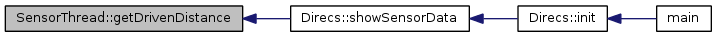Returns:
The heartbeat value which should look like high=5 or low=0 Volt.

Definition at line 1734 of file sensorThread.cpp.

Here is the caller graph for this function:int SensorThread::getIrSensorValue ( int sensor )
Parameters:
 sensor is the sensor number.
Returns:
The value of an infrared sensor.

Definition at line 713 of file sensorThread.cpp.

 int SensorThread::getMAmpere ( int motor )
Returns:
The measured motor sensor value in milli Ampere (mA).
Parameters:
 motor is the motor number.

Definition at line 668 of file sensorThread.cpp.

Here is the caller graph for this function:int SensorThread::getMotorSensorValue ( int sensor )
Parameters:
 sensor is the sensor number.
Returns:
The value of a motor sensor.

Definition at line 887 of file sensorThread.cpp.

 int SensorThread::getUsSensorValue ( int sensor )
Parameters:
 sensor is the sensor number.
Returns:
The value of an ultrasonic sensor.

Definition at line 862 of file sensorThread.cpp.

 float SensorThread::getVoltage ( int sensor )
Parameters:
 sensor is the sensor number and can be VOLTAGESENSOR1 or VOLTAGESENSOR2.
Returns:
The (already converted) voltage in Volt (V).

Definition at line 686 of file sensorThread.cpp.

Here is the call graph for this function: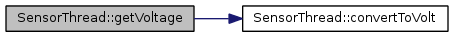Here is the caller graph for this function: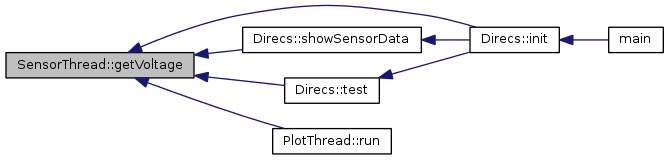void SensorThread::heartbeat ( unsigned char state )
signal

This signal is emitted every

Todo:
seconds, when a specific value from the microcontroller was received.
Gui::setLEDHeartbeat()
Logfile::writeHeartbeat()

Here is the caller graph for this function:void SensorThread::message ( QString text, bool CR = `true`, bool sayIt = `false`, bool addTimestamp = `true` )
signal

Emits a string to the GUI log / console.

Gui::appendLog()
Parameters:
 text is the message to be emitted CR is true when a CR/LF should be added at the end of the line (default) sayIt is true when the text for the log also should be spoken (default=false) addTimestamp If true, the a timestamp is added in front of the text. This parameter is optional.

Here is the caller graph for this function: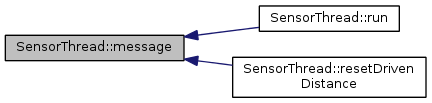private

Reads an axis value from the 3D compass from the microcontroller

Parameters:
 axis is the axis number (XAXIS, YAXIS or ZAXIS)
Returns:
true on success, false on error

Definition at line 1557 of file sensorThread.cpp.

Here is the call graph for this function: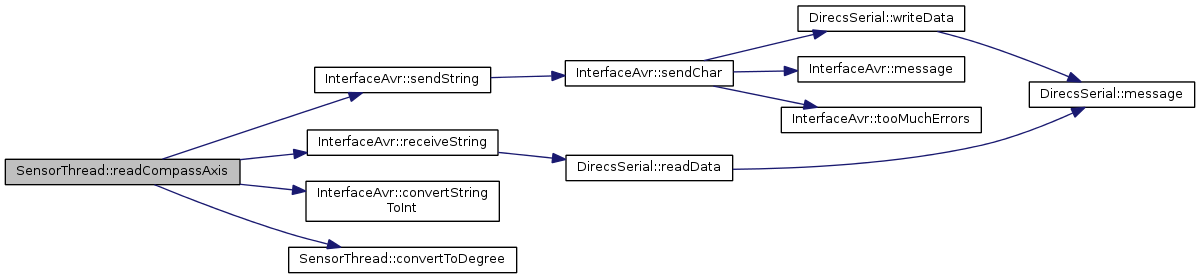private

Reads a contact value from the microcontroller (end contacts, when puhsing something or so)

Parameters:
 contact is the contact number (CONTACT1 to CONTACT4)
Returns:
true on success, false on error

Definition at line 1636 of file sensorThread.cpp.

Here is the call graph for this function:private

Reads a driven distance value from the microcontroller

Parameters:
 sensor is the sensor number (MOTORDISTANCE1 to MOTORDISTANCE2)
Returns:
true on success, false on error

Definition at line 1499 of file sensorThread.cpp.

Here is the call graph for this function: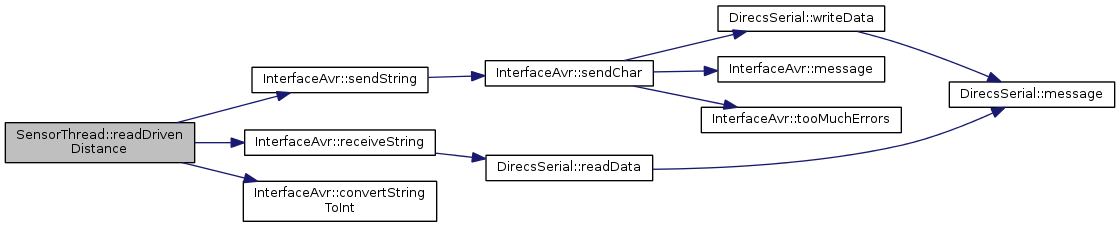private

Reads an infrared sensor value from the microcontroller

Parameters:
 sensor is the sensor number (SENSOR1 to SENSOR6)
Returns:
true on success, false on error

Definition at line 1108 of file sensorThread.cpp.

Here is the call graph for this function: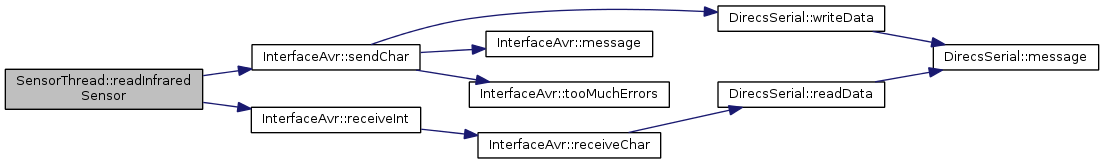private

Reads a motor sensor value from the microcontroller

Parameters:
 sensor is the sensor number (MOTORSENSOR1 to MOTORSENSOR4)
Returns:
true on success, false on error

Definition at line 1392 of file sensorThread.cpp.

Here is the call graph for this function: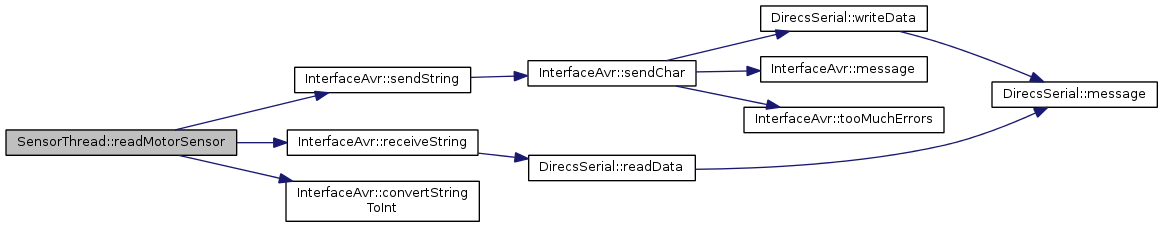private

Reads an ultrasonic sensor value from the microcontroller

Parameters:
 sensor is the sensor number (ULTRASONICSENSOR1)
Returns:
true on success, false on error

Definition at line 1298 of file sensorThread.cpp.

Here is the call graph for this function:private

Reads a voltage sensor value from the microcontroller

Parameters:
 sensor is the sensor number (VOLTAGESENSOR1 to VOLTAGESENSOR2)
Returns:
true on success, false on error

Definition at line 1334 of file sensorThread.cpp.

Here is the call graph for this function: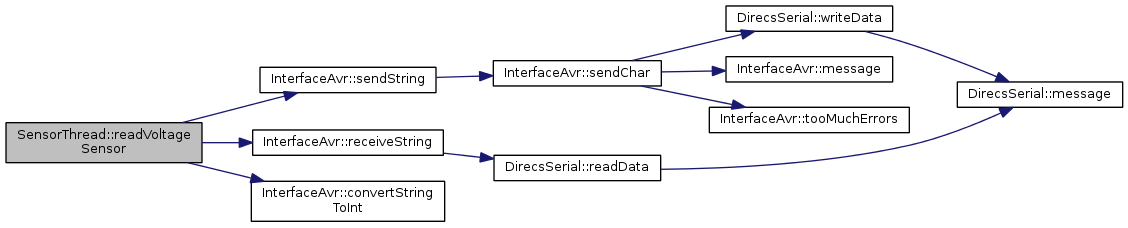Here is the caller graph for this function:bool SensorThread::resetDrivenDistance ( int sensor )
slot

Resets the driven distance to 0.

Parameters:
 sensor is the sensor number.
Returns:
true on success, false on error

Definition at line 786 of file sensorThread.cpp.

Here is the call graph for this function: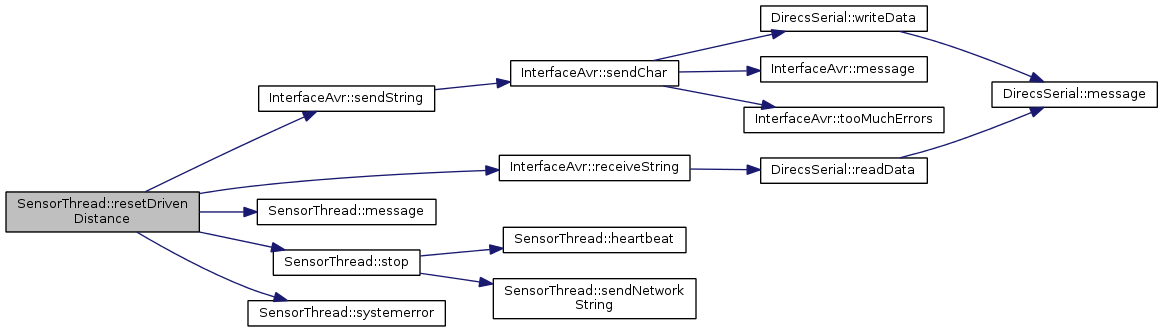virtual

Todo:
implement reading of motor sensors 3 and 4 !

Definition at line 169 of file sensorThread.cpp.

Here is the call graph for this function: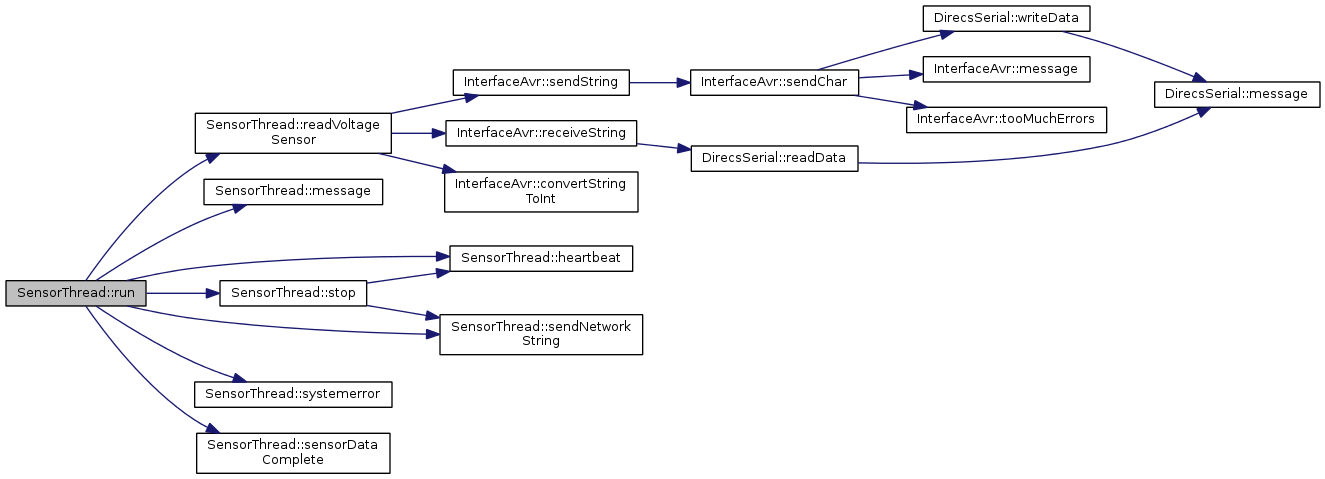void SensorThread::sendNetworkString ( QString text )
signal

Sends a string over the network.

Here is the caller graph for this function:signal

This signal is emitted when all sensors were read.

Direcs::showSensorData()

Here is the caller graph for this function: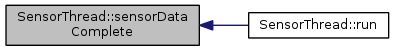void SensorThread::setCompassState ( bool state )
slot

This slots takes the compass circuit state, to know if the compas module is connected or not. When the class knows this it is unnecessary to communicate with the interface.

Parameters:
 state can be true or false

Definition at line 1026 of file sensorThread.cpp.

 void SensorThread::setRobotState ( bool state )
slot

This slots takes the robot (circuit) state, to know if the robot is ON or OFF. When the class knows this, unnecessary communication with the interface can be avoided.

Parameters:
 state can be ON or OFF

Definition at line 1020 of file sensorThread.cpp.

 void SensorThread::setSimulationMode ( bool state )
slot

This slot enables or disables the simulation mode. In the simulation mode all sensor values are set to a fixed value. No real sensor values are read from the robot, when set to true.

Parameters:
 state can be true or false.
Gui::simulate()

Definition at line 904 of file sensorThread.cpp.

Here is the call graph for this function: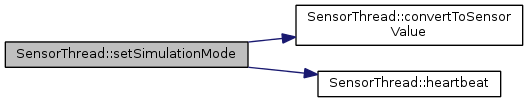Definition at line 156 of file sensorThread.cpp.

Here is the call graph for this function: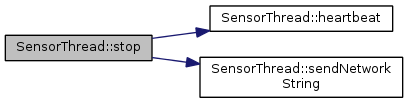Here is the caller graph for this function: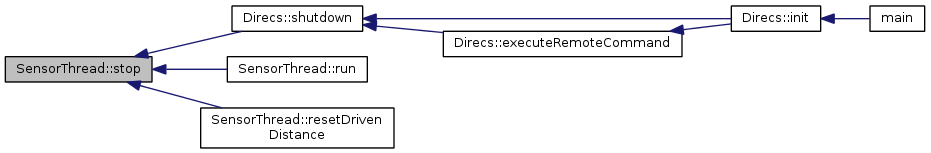void SensorThread::systemerror ( int errorlevel )
signal

Emits a emergency signal for letting other modules know that we have a massive sensor error. So in that case an emergency stop or so could be initiated.

Parameters:
 errorlevel needs to be defined. Temporariliy we use -2 in case of error in this thread.

Here is the caller graph for this function: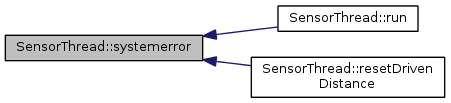## Member Data Documentation

staticprivate

Definition at line 419 of file sensorThread.h.

private

Definition at line 286 of file sensorThread.h.

private

Definition at line 292 of file sensorThread.h.

 const unsigned char SensorThread::CONTACT1 = 0
staticprivate
```               For motor or robot "OFF"
```

Give the contacts for the pan tilt cam end positions some nice names

Definition at line 410 of file sensorThread.h.

 const unsigned char SensorThread::CONTACT2 = 1
staticprivate

Definition at line 411 of file sensorThread.h.

 const unsigned char SensorThread::CONTACT3 = 2
staticprivate

Definition at line 412 of file sensorThread.h.

 const unsigned char SensorThread::CONTACT4 = 3
staticprivate

Definition at line 413 of file sensorThread.h.

 const unsigned char SensorThread::CONTACTARRAYSIZE = 4
staticprivate

Definition at line 421 of file sensorThread.h.

private

Definition at line 422 of file sensorThread.h.

 const unsigned char SensorThread::CONVERSIONFACTORMOTORSENSOR = 29
staticprivate

Defines the conversion factor for the motor sensors to convert the sensor value in a "real world" value. For example, a measured sensor value for a motor sensor is 100, this multiplied with a conversion factor 29 results in 290 mA.

Definition at line 377 of file sensorThread.h.

staticprivate

Defines the conversion factor for the voltage sensors to convert the sensor value in a "real world" value. For example, a measured sensor value for a voltage sensor is 685, this divided by a conversion factor 57.0 results in 12 V. Lead-Gel: 4095 / 13.2 Volt = 310.22727272 4095 / 26.4 Volt = 155.11363636 LiPo: 4095 / 16.8 Volt = 243.75 (4S) 4095 / 25.2 Volt = 162.50 (6S)

Definition at line 389 of file sensorThread.h.

staticprivate

Definition at line 390 of file sensorThread.h.

private

Definition at line 363 of file sensorThread.h.

 const short int SensorThread::DRIVENDISTANCE1 = 0
staticprivate

Definition at line 365 of file sensorThread.h.

 const short int SensorThread::DRIVENDISTANCE2 = 1
staticprivate

Definition at line 366 of file sensorThread.h.

 const unsigned char SensorThread::DRIVENDISTANCEARRAYSIZE = 2
staticprivate

Definition at line 362 of file sensorThread.h.

 const unsigned char SensorThread::GREEN = 2
staticprivate

Definition at line 460 of file sensorThread.h.

private

Definition at line 398 of file sensorThread.h.

private

Since a heartbeat can only high or low. we store only 1 value in this array. But we need an array for the plotThread.

Definition at line 371 of file sensorThread.h.

private

Definition at line 288 of file sensorThread.h.

private

Definition at line 327 of file sensorThread.h.

 const unsigned char SensorThread::IRSENSORARRAYSIZE = 40
staticprivate
Todo:
array mit 129 Werten statt 8 für 8 Sensoren !!!

defines the size of the iRDistance[] array !!

Definition at line 326 of file sensorThread.h.

private

Definition at line 323 of file sensorThread.h.

 const unsigned char SensorThread::LEDOFF = 0
staticprivate

This is for the

heartbeat signal (the GUI LED colors)

Definition at line 458 of file sensorThread.h.

staticprivate

Definition at line 416 of file sensorThread.h.

staticprivate

Definition at line 337 of file sensorThread.h.

 const short int SensorThread::MOTORSENSOR1 = 0
staticprivate

Definition at line 355 of file sensorThread.h.

 const short int SensorThread::MOTORSENSOR2 = 1
staticprivate

Definition at line 356 of file sensorThread.h.

 const short int SensorThread::MOTORSENSOR3 = 2
staticprivate

Definition at line 357 of file sensorThread.h.

 const short int SensorThread::MOTORSENSOR4 = 3
staticprivate

Definition at line 358 of file sensorThread.h.

 const unsigned char SensorThread::MOTORSENSORARRAYSIZE = 4
staticprivate

Defines the size of the motorSensorValue[] array This es equal to the number of motor sensors.

Definition at line 352 of file sensorThread.h.

private

Definition at line 353 of file sensorThread.h.

mutableprivate

this will contain the name of this class at runtime for debug messages

Definition at line 287 of file sensorThread.h.

staticprivate

For motor or robot "ON"

Definition at line 405 of file sensorThread.h.

staticprivate

Definition at line 404 of file sensorThread.h.

staticprivate
Todo:
why int instead of char? Where else?

Definition at line 446 of file sensorThread.h.

staticprivate

Definition at line 447 of file sensorThread.h.

staticprivate

Definition at line 448 of file sensorThread.h.

staticprivate

Definition at line 450 of file sensorThread.h.

staticprivate

Definition at line 451 of file sensorThread.h.

staticprivate

Definition at line 452 of file sensorThread.h.

staticprivate

Definition at line 453 of file sensorThread.h.

staticprivate

Definition at line 440 of file sensorThread.h.

staticprivate

Definition at line 441 of file sensorThread.h.

staticprivate

Definition at line 437 of file sensorThread.h.

staticprivate

Definition at line 438 of file sensorThread.h.

staticprivate

Definition at line 427 of file sensorThread.h.

staticprivate

Definition at line 435 of file sensorThread.h.

staticprivate

Definition at line 428 of file sensorThread.h.

staticprivate

Definition at line 429 of file sensorThread.h.

staticprivate

Definition at line 430 of file sensorThread.h.

staticprivate

Definition at line 431 of file sensorThread.h.

staticprivate

Definition at line 432 of file sensorThread.h.

staticprivate

Definition at line 433 of file sensorThread.h.

staticprivate

Definition at line 434 of file sensorThread.h.

 const unsigned char SensorThread::RED = 1
staticprivate

Definition at line 459 of file sensorThread.h.

 const short int SensorThread::RESET_MOTOR_DISTANCE1 = 32
staticprivate

Definition at line 443 of file sensorThread.h.

 const short int SensorThread::RESET_MOTOR_DISTANCE2 = 33
staticprivate
Todo:
why int instead of char? Where else?

Definition at line 444 of file sensorThread.h.

staticprivate

Definition at line 417 of file sensorThread.h.

private

Definition at line 291 of file sensorThread.h.

 const short int SensorThread::SENSOR1 = 1
staticprivate

Definition at line 303 of file sensorThread.h.

 const short int SensorThread::SENSOR16 = 256
staticprivate

Definition at line 313 of file sensorThread.h.

 const short int SensorThread::SENSOR2 = 2
staticprivate

Definition at line 304 of file sensorThread.h.

 const short int SensorThread::SENSOR3 = 4
staticprivate

Definition at line 305 of file sensorThread.h.

 const short int SensorThread::SENSOR4 = 8
staticprivate

Definition at line 306 of file sensorThread.h.

 const short int SensorThread::SENSOR5 = 16
staticprivate

Definition at line 307 of file sensorThread.h.

 const short int SensorThread::SENSOR6 = 32
staticprivate

Definition at line 308 of file sensorThread.h.

 const short int SensorThread::SENSOR7 = 64
staticprivate

Definition at line 309 of file sensorThread.h.

 const short int SensorThread::SENSOR8 = 128
staticprivate

Definition at line 310 of file sensorThread.h.

private

Definition at line 290 of file sensorThread.h.

private

Definition at line 289 of file sensorThread.h.

staticprivate

Definition at line 296 of file sensorThread.h.

staticprivate

Definition at line 418 of file sensorThread.h.

 const short int SensorThread::ULTRASONICSENSOR1 = 0
staticprivate

Definition at line 346 of file sensorThread.h.

 const unsigned char SensorThread::USSENSORARRAYSIZE = 1
staticprivate

theoratically 5 Volt for heartbeat "high"

Gui::initPlot Defines the size of the usSensorValue[] array This es equal to the number of ultrasonic sensors.

Definition at line 343 of file sensorThread.h.

private

Definition at line 344 of file sensorThread.h.

 const short int SensorThread::VOLTAGESENSOR1 = 0
staticprivate

Definition at line 334 of file sensorThread.h.

 const short int SensorThread::VOLTAGESENSOR2 = 1
staticprivate

Definition at line 335 of file sensorThread.h.

private

for storing the voltage values from the accumulators (power supply)

Definition at line 332 of file sensorThread.h.

private

The x, y, z axis value from the 3D magnetic sensor and the heading

Definition at line 395 of file sensorThread.h.

 const short int SensorThread::XAXIS = 0
staticprivate

Definition at line 400 of file sensorThread.h.

private

Definition at line 396 of file sensorThread.h.

 const short int SensorThread::YAXIS = 1
staticprivate

Definition at line 401 of file sensorThread.h.